### Home > AC > Chapter 13 > Lesson 13.IF2-S > Problem6-36

6-36.
1. Consider the function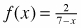. Homework Help ✎

1. What is f(7)?

2. What is the domain of f(x)?

3. If g(x) = 2x + 5, what is g(3)?

4. Now use the output of g(3) as the input for f to calculate f(g(3)).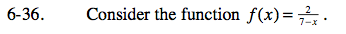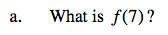Undefined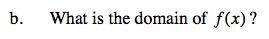x ≠ 7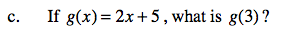11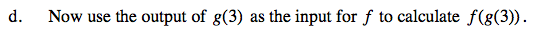Substitute the value of g(3) for x in f(x).